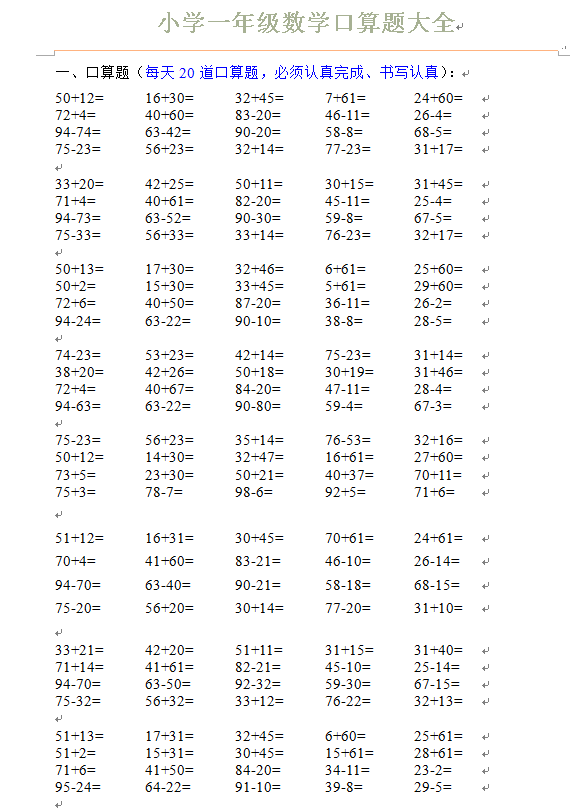50+12=          16+30=          32+45=          7+61=             24+60=

72+4=             40+60=          83-20=         46-11=           26-4=

94-74=            63-42=        90-20=            58-8=            68-5=

75-23=          56+23=          32+14=          77-23=             31+17=